In:=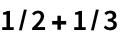Out=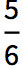In:=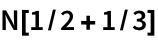Out=If theres any decimal number in your input, the Wolfram Language will automatically give you an approximate answer.
The presence of a decimal number makes the result be approximate:
 In:=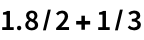Out=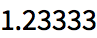In:=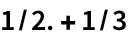Out=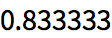Heres 2 raised to the power 1000:
 In:=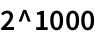Out=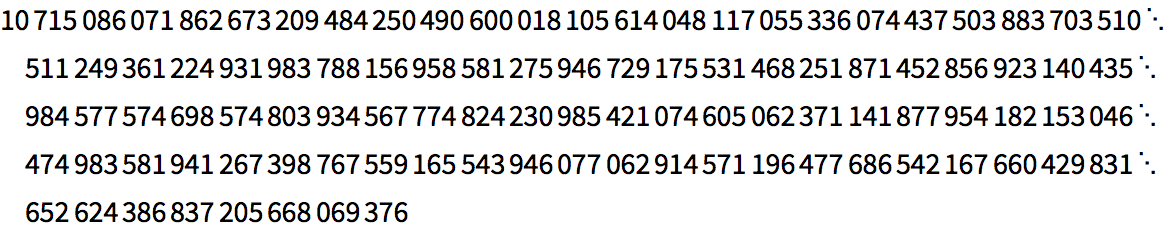Get a numerical approximation:
 In:=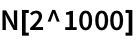Out=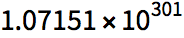Enter a number in scientific notation:
 In:=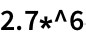Out=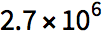Get a numerical approximation to π:
 In:=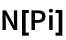Out=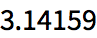Compute 250 digits of π:
 In:=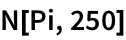Out=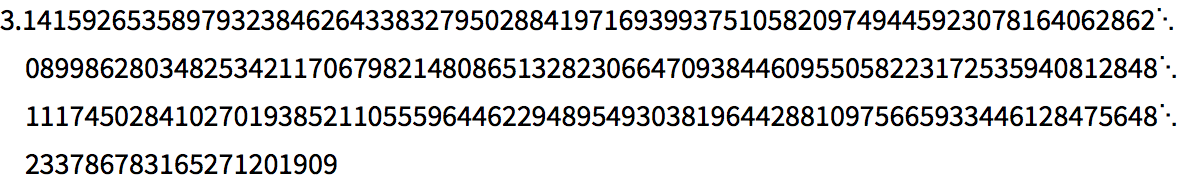Generate a random real number in the range 0 to 10:
 In:=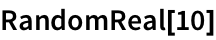Out=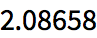Generate 5 random real numbers:
 In:=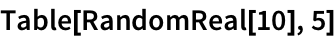Out=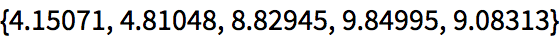An alternative way to ask for 5 random real numbers:
 In:=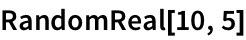Out=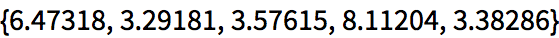In:=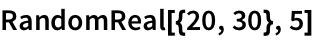Out=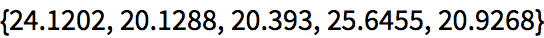The Wolfram Language has a huge range of mathematical functions built in, from basic to very sophisticated.
 In:=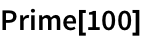Out=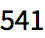Find the millionth prime number:
 In:=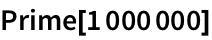Out=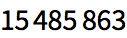Plot the first 50 primes:
 In:=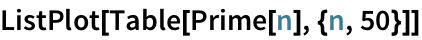Out=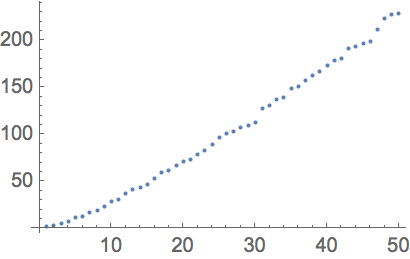Three functions common in many practical situations are Sqrt (square root), Log10 (logarithm to base 10) and Log (natural logarithm).
The square root of 16 is 4:
 In:=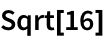Out=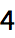If you dont ask for a numerical approximation, youll get an exact formula:
 In:=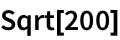Out=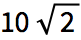N gives a numerical approximation:
 In:=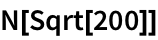Out=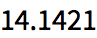Logarithms are often useful when youre dealing with numbers that have a wide range of sizes. Lets plot the masses of the planets. With ListPlot one cant tell anything about the planets before Jupiter. But ListLogPlot shows the relative sizes much more clearly.
Make an ordinary ListPlot of the masses of the planets:
 In:=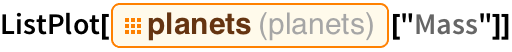Out=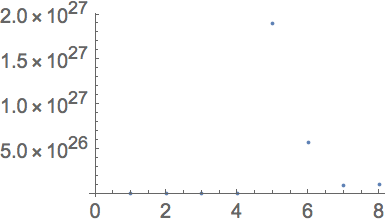In:=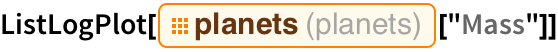Out=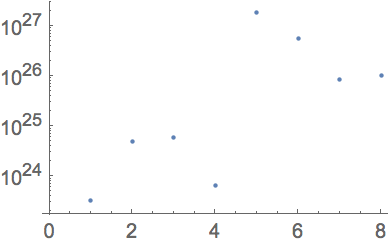Abs effectively just drops minus signs:
 In:=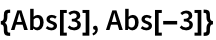Out=Next theres Round, which rounds to the nearest whole number.
Round rounds to the nearest whole number:
 In:=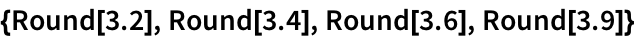Out=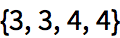Another function thats very useful is Mod. Lets say youre counting up minutes in an hour. When you reach 60, youll want to start again from 0. Thats what Mod lets you do.
Compute a sequence of numbers mod 60:
 In:=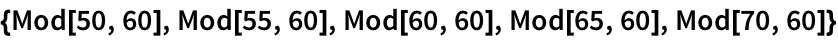Out=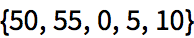N[expr] numerical approximation Pi the number π (pi)≃3.14 Sqrt[x] square root Log10[x] logarithm to base 10 Log[x] natural logarithm (ln) Abs[x] absolute value (drop minus signs) Round[x] round to nearest integer Prime[n] nth prime number Mod[x,n] modulo (“clock arithmetic”) RandomReal[max] random real number between 0 and max RandomReal[max,n] list of n random real numbers ListLogPlot[data] plot on a logarithmic scale
23.1Find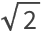to 500-digit precision. »
Expected output:
 Out[]=Answer & check your solution
23.2Generate 10 random real numbers between 0 and 1. »
Sample expected output:
 Out[]=Answer & check your solution
23.3Make a plot of 200 points with random real x and y coordinates between 0 and 1. »
Sample expected output:
 Out[]=Answer & check your solution
23.4Create a random walk using AnglePath and 1000 random real numbers between 0 and 2π»
Sample expected output:
 Out[]=Answer & check your solution
23.5Make a table of Mod[n^2, 10] for n from 0 to 30. »
Expected output:
 Out[]=Answer & check your solution
23.6Make a line plot of Mod[n^n, 10] for n from 1 to 100. »
Expected output:
 Out[]=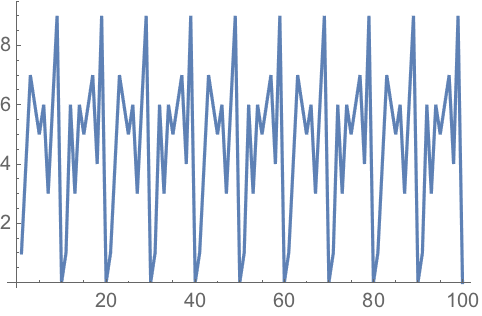Answer & check your solution
23.7Make a table of the first 10 powers of π, rounded to integers. »
Expected output:
 Out[]=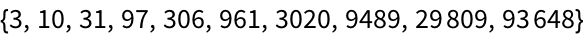Answer & check your solution
23.8Make a graph by connecting n with Mod[n^2, 100] for n from 0 to 99. »
Expected output:
 Out[]=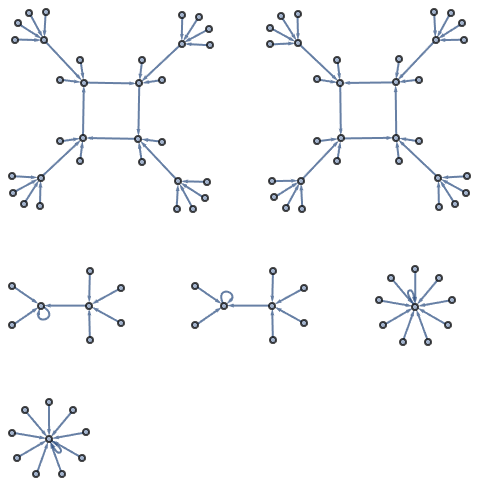Answer & check your solution
Sample expected output:
 Out[]=Answer & check your solution
Expected output:
 Out[]=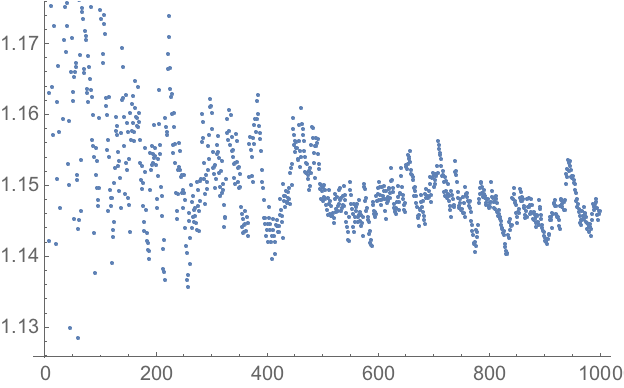Answer & check your solution
23.11Make a line plot of the differences between successive primes up to 100. »
Expected output:
 Out[]=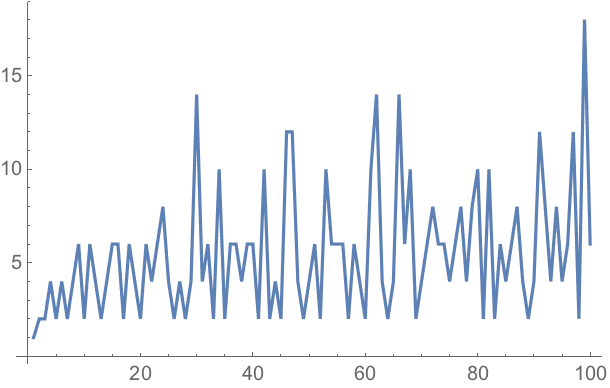Answer & check your solution
23.12Generate a sequence of 20 middle C notes with random durations between 0 and 0.5 seconds. »
Sample expected output:
 Out[]=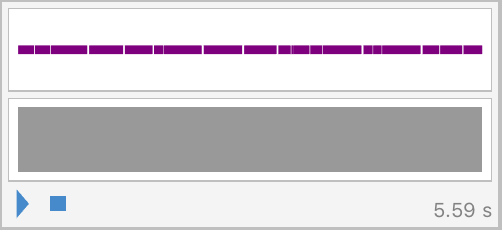Answer & check your solution
23.13Make an array plot of Mod[i, j] for i and j up to 50. »
Expected output:
 Out[]=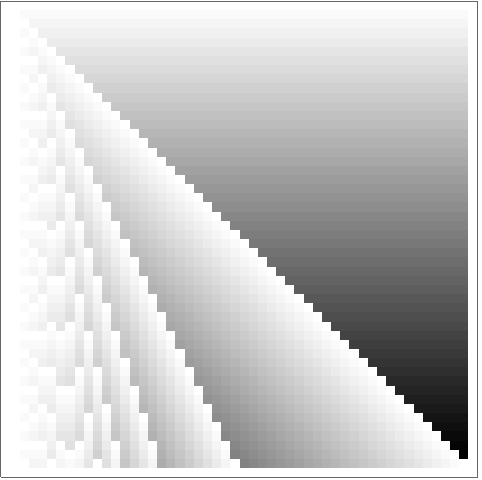Answer & check your solution
23.14Make a list for n from 2 to 10 of array plots for x and y up to 50 of x^y mod n»
Expected output:
 Out[]=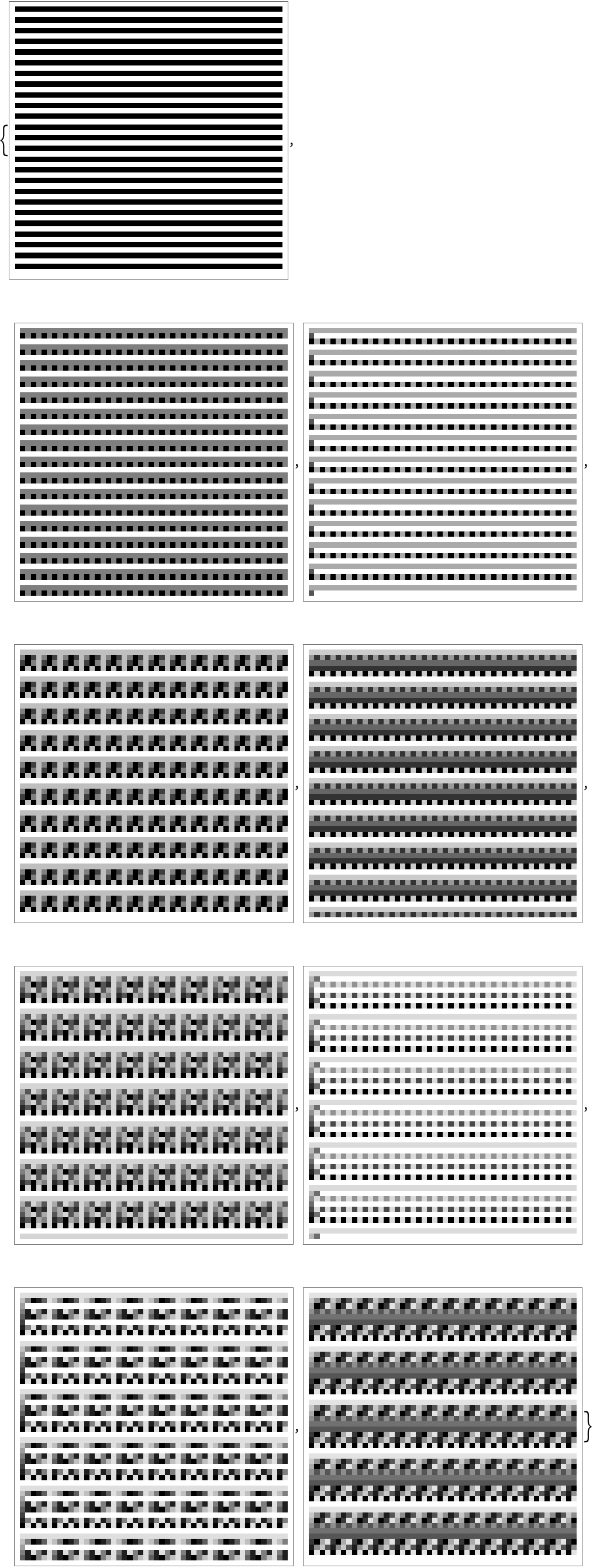Answer & check your solution
+23.1Use Round to compute the fractional part of π to 50 digits. »
Expected output:
 Out[]=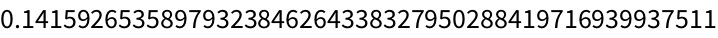Answer & check your solution
+23.2Find the sum of the first 1000 prime numbers. »
Expected output:
 Out[]=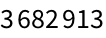Answer & check your solution
+23.3Make a list of the first 100 primes modulo 4. »
Expected output:
 Out[]=Answer & check your solution
+23.4Make a list of the first 10000 primes modulo 4, multiply them by 90° and create an angle path from them. »
Expected output:
 Out[]=Answer & check your solution
From standard school math, ones like Sin, Cos, ArcTan, Exp, as well as GCD, Factorial, Fibonacci. From physics and engineering and higher math, ones like Gamma (gamma function), BesselJ (Bessel function), EllipticK (elliptic integral), Zeta (Riemann zeta function), PrimePi, EulerPhi. From statistics, ones like Erf, NormalDistribution, ChiSquareDistribution. Hundreds of functions altogether.
Its the total number of decimal digits quoted in the number. N[100/3, 5] gives 33.333, which has 5 digits of precision. The number 100/3 is exact; N[100/3, 5] approximates it to 5-digit precision.
What does the at the end of each line in a long number mean?
Its there to show that the number continues onto the next linelike a hyphen in text.
Of course. The symbol I (capital i) represents the square root of 1.
Why does N[1.5/7, 100] not give me a 100-digit result?
Because 1.5 is an approximate number with much less than 100-digit precision. N[15/70, 100] will for example give a 100-digit-precision number.